# How to Calculate and Solve for Ratio of Load of Fibre to Matrix | Composites

The ratio of load of fibre to matrix is illustrated by the image below.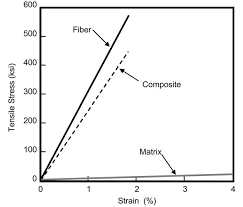To compute for ratio of load of fibre to matrix, four essential parameters are needed and these parameters are Elastic Modulus of the Fibre (Ef), Elastic Modulus of the Matrix (Em), Volume Fraction of the Fibre (Vf) and Volume Fraction of the Matrix (Vm).

The formula for calculating ratio of load of fibre to matrix:

F(f/m) = EfVf/EmVm

Where:

F(f/m) = Ratio of Load of Fibre to Matrix
Ef = Elastic Modulus of the Fibre
Em = Elastic Modulus of the Matrix
Vf = Volume Fraction of the Fibre
Vm = Volume Fraction of the Matrix

Let’s solve an example;
Find the ratio of load of fibre to matix when the elastic modulus of the fibre is 14, the elastic modulus of the matrix is 10, the volume fraction of the fibre is 6 and the volume fraction of the matrix is 2.

This implies that;

Ef = Elastic Modulus of the Fibre = 14
Em = Elastic Modulus of the Matrix = 10
Vf = Volume Fraction of the Fibre = 6
Vm = Volume Fraction of the Matrix = 2

F(f/m) = EfVf/EmVm
F(f/m) = (14)(6)/(10)(2)
F(f/m) = (84)/(20)
F(f/m) = 4.2

Therefore, the ratio of load of fibre to matrix is 4.2.

Calculating the Elastic Modulus of the Fibre when the Ratio of Load of Fibre to Matrix, the Elastic Modulus of the Matrix, the Volume Fraction of the Fibre and the Volume Fraction of the Matrix is Given.

Ef = F(f/m) x EmVm / Vf

Where:

Ef = Elastic Modulus of the Fibre
F(f/m) = Ratio of Load of Fibre to Matrix
Em = Elastic Modulus of the Matrix
Vf = Volume Fraction of the Fibre
Vm = Volume Fraction of the Matrix

Let’s solve an example;
Find the elastic modulus of the fibre when the ratio of load of fibre to matrix is 20, the elastic modulus of the matrix is 4, the volume fraction of the matrix is 2 and the volume fraction of the fibre is 12.

This implies that;

F(f/m) = Ratio of Load of Fibre to Matrix = 20
Em = Elastic Modulus of the Matrix = 4
Vf = Volume Fraction of the Fibre = 12
Vm = Volume Fraction of the Matrix = 2

Ef = F(f/m) x EmVm / Vf
Ef = 20 x (4)(2) / 12
Ef = 20 x 8 / 12
Ef = 160 / 12
Ef = 13.33

Therefore, the elastic modulus of the fibre is 13.33

Calculating the Elastic Modulus of the Matrix when the Ratio of Load of Fibre to Matrix, the Elastic Modulus of the Fibre, the Volume Fraction of the Fibre and the Volume Fraction of the Matrix is Given.

Em = EfVf / F(f/m) x Vm

Where:

Em = Elastic Modulus of the Matrix
F(f/m) = Ratio of Load of Fibre to Matrix
Ef = Elastic Modulus of the Fibre
Vf = Volume Fraction of the Fibre
Vm = Volume Fraction of the Matrix

Let’s solve an example;
Find the elastic modulus of the matrix when the ratio of load of fibre to matrix is 18, the elastic modulus of the fibre is 12, the volume fraction of the fibre is 14 and the volume fraction of the matrix is 8.

This implies that;

F(f/m) = Ratio of Load of Fibre to Matrix = 18
Ef = Elastic Modulus of the Fibre = 12
Vf = Volume Fraction of the Fibre = 14
Vm = Volume Fraction of the Matrix = 8

Em = EfVf / F(f/m) x Vm
Em = (12)(14) / 18 x 8
Em = 168 / 144
Em = 1.167

Therefore, the elastic modulus of the matrix is 1.167

Calculating the Volume Fraction of the Matrix when the Ratio of Load of Fibre to Matrix, the Elastic Modulus of the Fibre, the Elastic Modulus of the Matrix and the Volume Fraction of the Fibre is Given.

Vf = F(f/m) x EmVm / Ef

Where:

Vf = Volume Fraction of the Fibre
F(f/m) = Ratio of Load of Fibre to Matrix
Ef = Elastic Modulus of the Fibre
Em = Elastic Modulus of the Matrix
Vm = Volume Fraction of the Matrix

Let’s solve an example;
Find the volume fraction of the fibre when the ratio of load of fibre to matrix is 7, the elastic modulus of the fibre is 20, the elastic modulus of the matrix is 4 and the volume fraction of the matrix is 6.

This implies that;

F(f/m) = Ratio of Load of Fibre to Matrix = 7
Ef = Elastic Modulus of the Fibre = 20
Em = Elastic Modulus of the Matrix = 4
Vm = Volume Fraction of the Matrix = 6

Vf = F(f/m) x EmVm / Ef
Vf = 7 x (4)(6) / 20
Vf = 168 / 20
Vf = 8.4

Therefore, the volume fraction of the fibre is 8.4

Calculating the Volume Fraction of the Fibre when the Ratio of Load of Fibre to Matrix, the Elastic Modulus of the Fibre, the Elastic Modulus of the Matrix and the Volume Fraction of the Matrix is Given.

Vm = EfVf / F(f/m) x Em

Where:

Vm = Volume Fraction of the Matrix
F(f/m) = Ratio of Load of Fibre to Matrix
Ef = Elastic Modulus of the Fibre
Em = Elastic Modulus of the Matrix
Vf = Volume Fraction of the Fibre

Let’s solve an example;
Find the volume fraction of the matrix when the ratio of load of fibre to matrix is 10, the elastic modulus of the fibre is 16, the elastic modulus of the matrix is 6 and the volume fraction of the fibre is 8.

This implies that;

F(f/m) = Ratio of Load of Fibre to Matrix = 10
Ef = Elastic Modulus of the Fibre = 16
Em = Elastic Modulus of the Matrix = 6
Vf = Volume Fraction of the Fibre = 8

Vm = EfVf / F(f/m) x Em
Vm = (16)(8) / 10 x 6
Vm = 128 / 60
Vm = 2.13

Therefore, the volume fraction of the matrix is 2.13

Nickzom Calculator – The Calculator Encyclopedia is capable of calculating the ratio of load of fibre to matrix.

To get the answer and workings of the ratio of load of fibre to matrix using the Nickzom Calculator – The Calculator Encyclopedia. First, you need to obtain the app.

You can get this app via any of these means:

You can also try the demo version via https://www.nickzom.org/calculator

Apple (Paid) – https://itunes.apple.com/us/app/nickzom-calculator/id1331162702?mt=8
Once, you have obtained the calculator encyclopedia app, proceed to the Calculator Map, then click on Materials and Metallurgical under Engineering.Now, Click on Composites under Materials and MetallurgicalNow, Click on Ratio of Load of Fibre to Matrix under Composites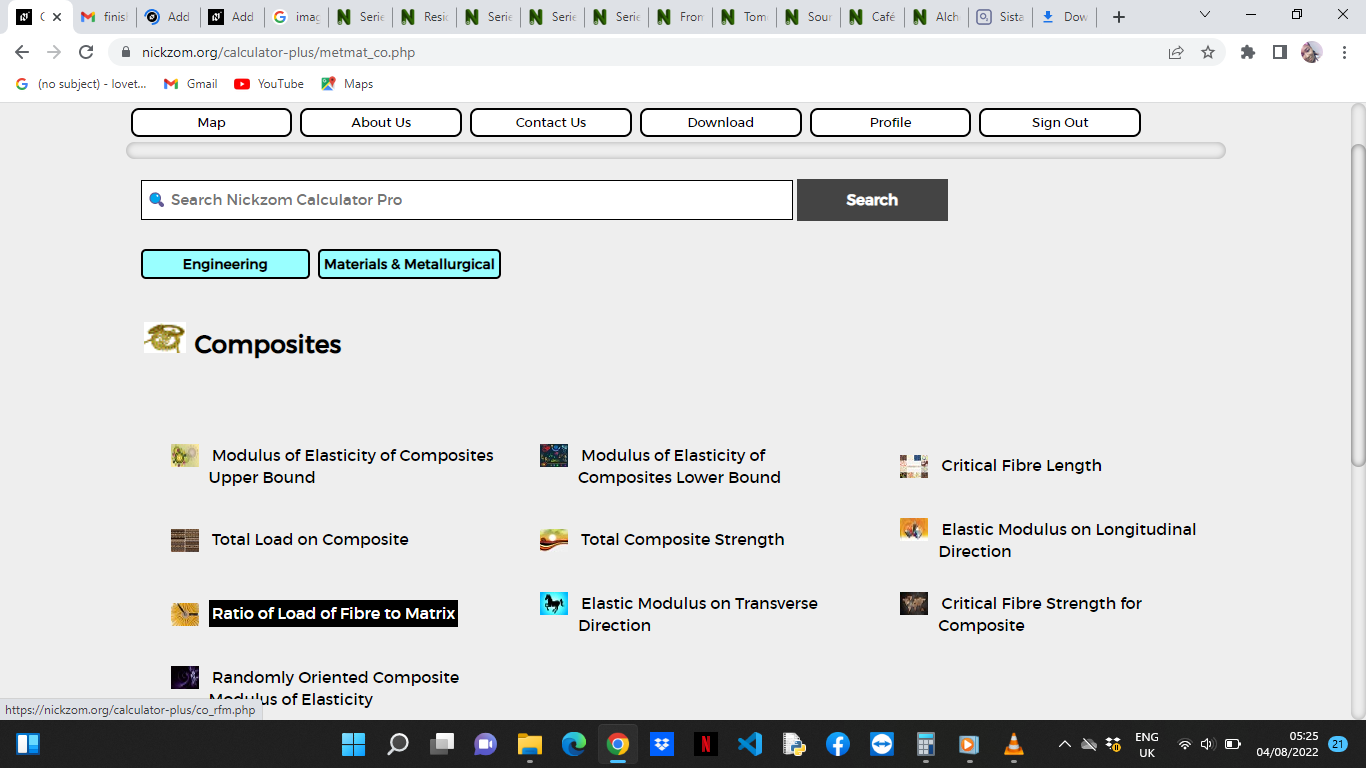The screenshot below displays the page or activity to enter your values, to get the answer for the ratio of load of fibre to matrix according to the respective parameter which is the Elastic Modulus of the Fibre (Ef), Elastic Modulus of the Matrix (Em), Volume Fraction of the Fibre (Vf) and Volume Fraction of the Matrix (Vm).Now, enter the values appropriately and accordingly for the parameters as required by the Elastic Modulus of the Fibre (Ef) is 14, Elastic Modulus of the Matrix (Em) is 10, Volume Fraction of the Fibre (Vfis 6 and Volume Fraction of the Matrix (Vm) is 2.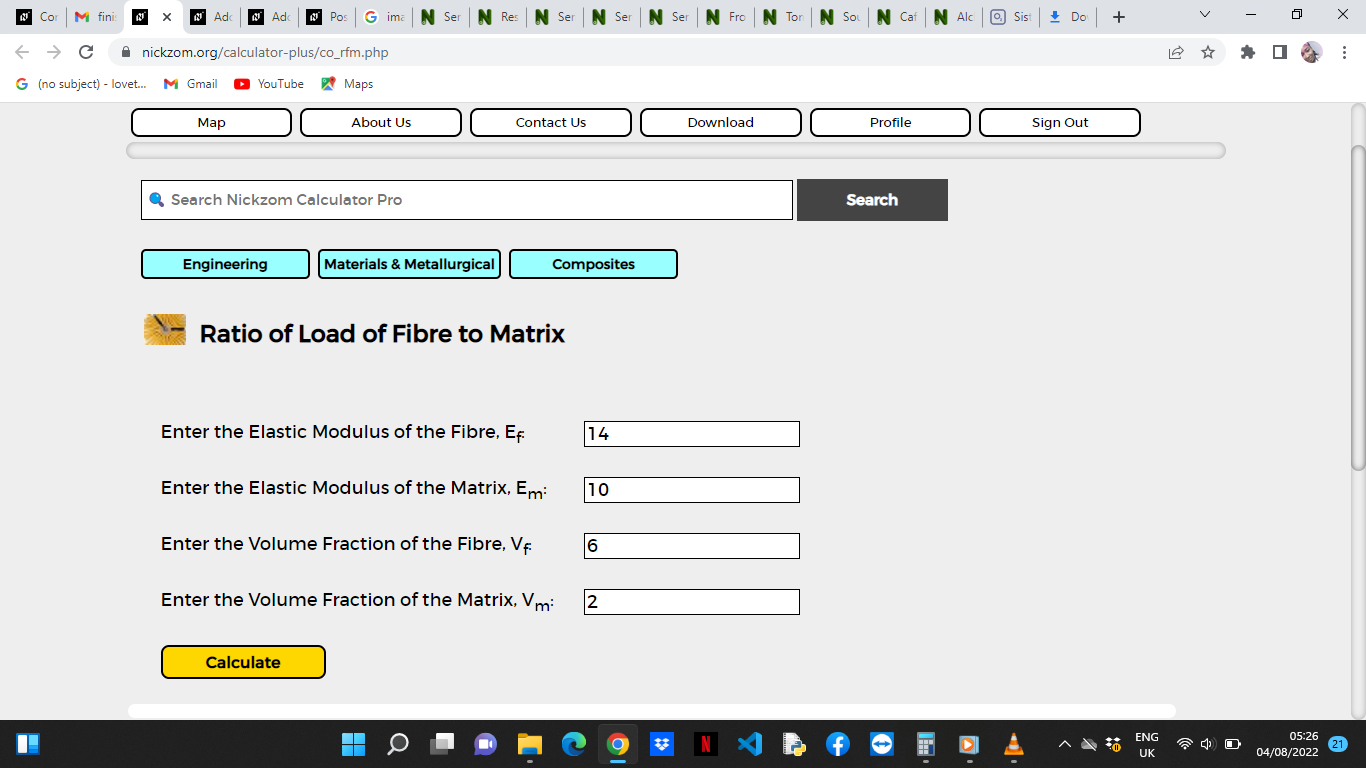Finally, Click on Calculate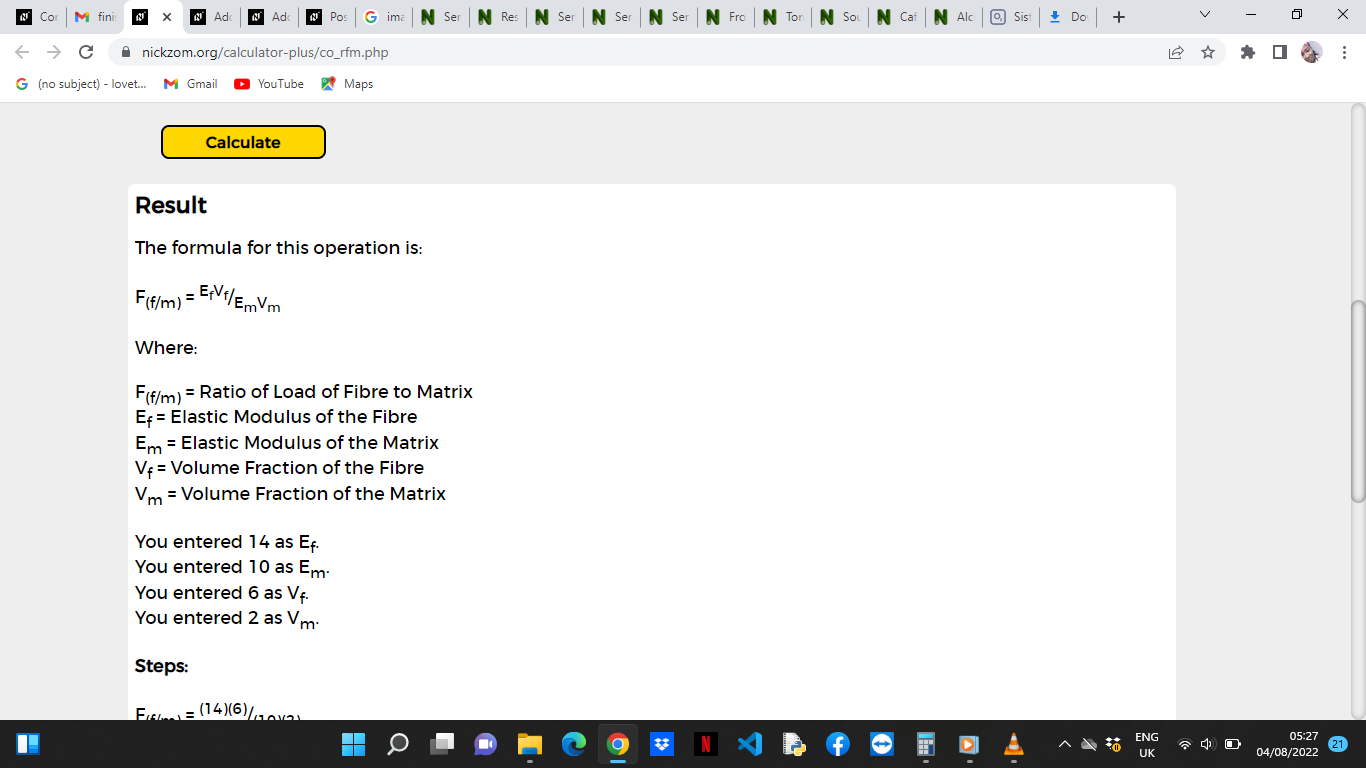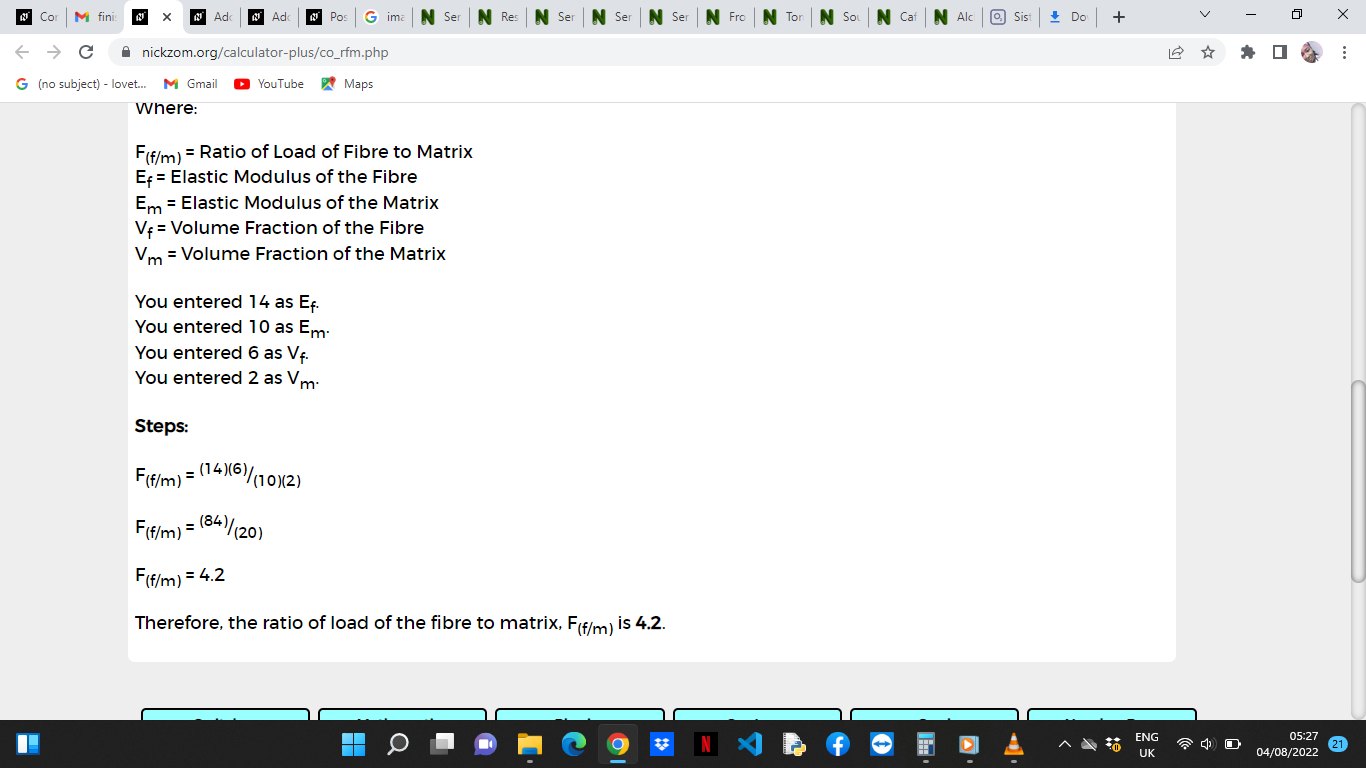As you can see from the screenshot above, Nickzom Calculator– The Calculator Encyclopedia solves for the ratio of load of fibre to matrix and presents the formula, workings and steps too.